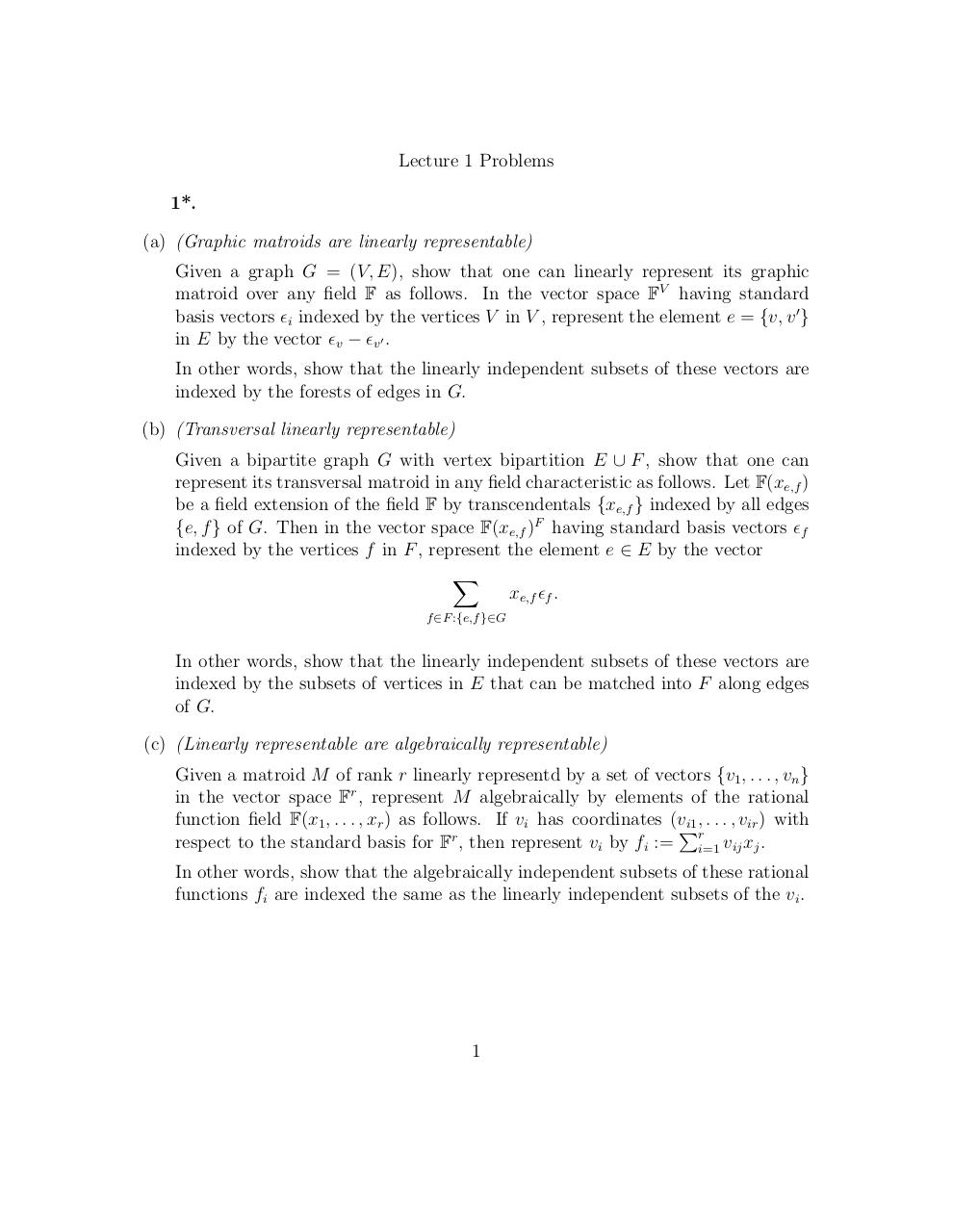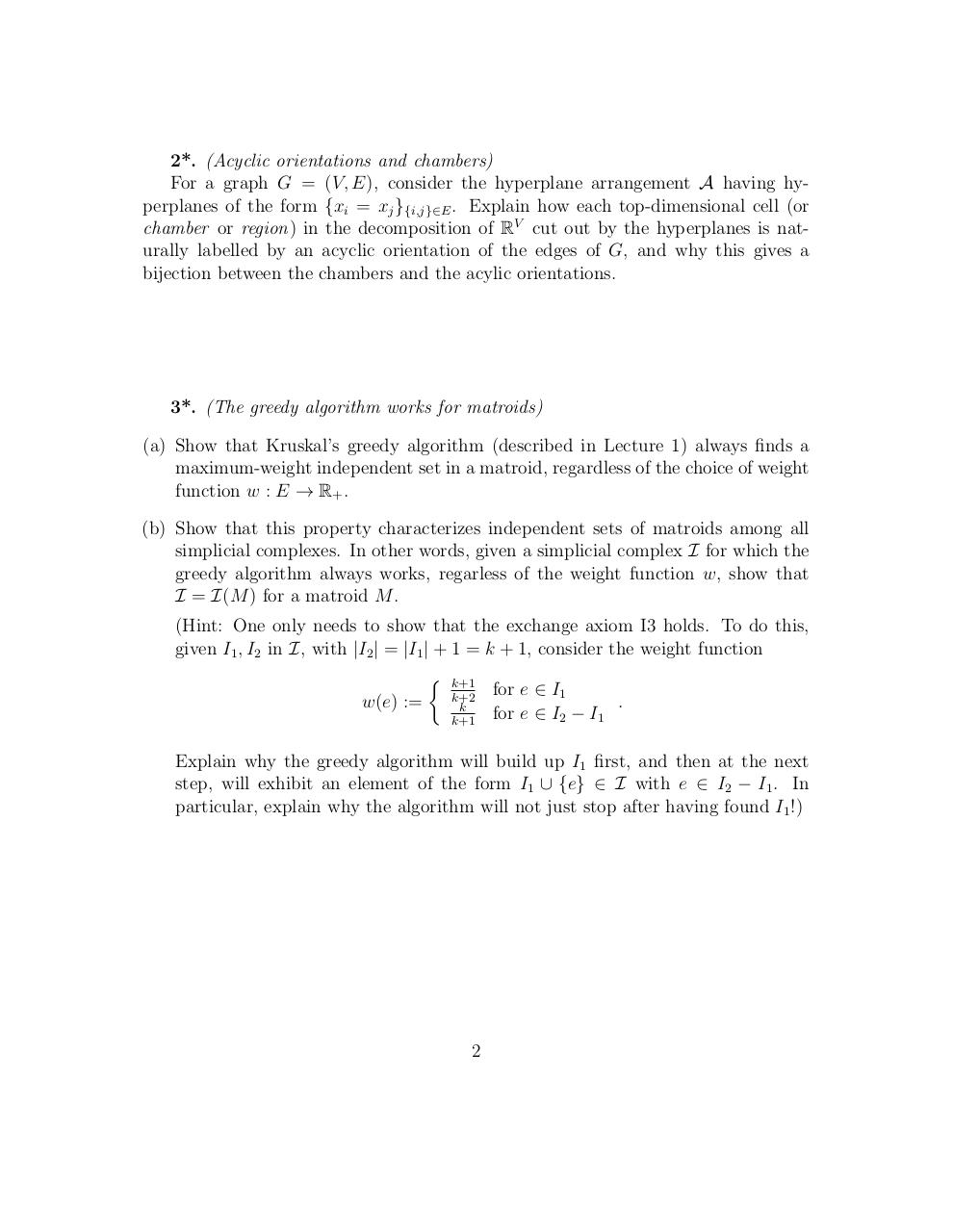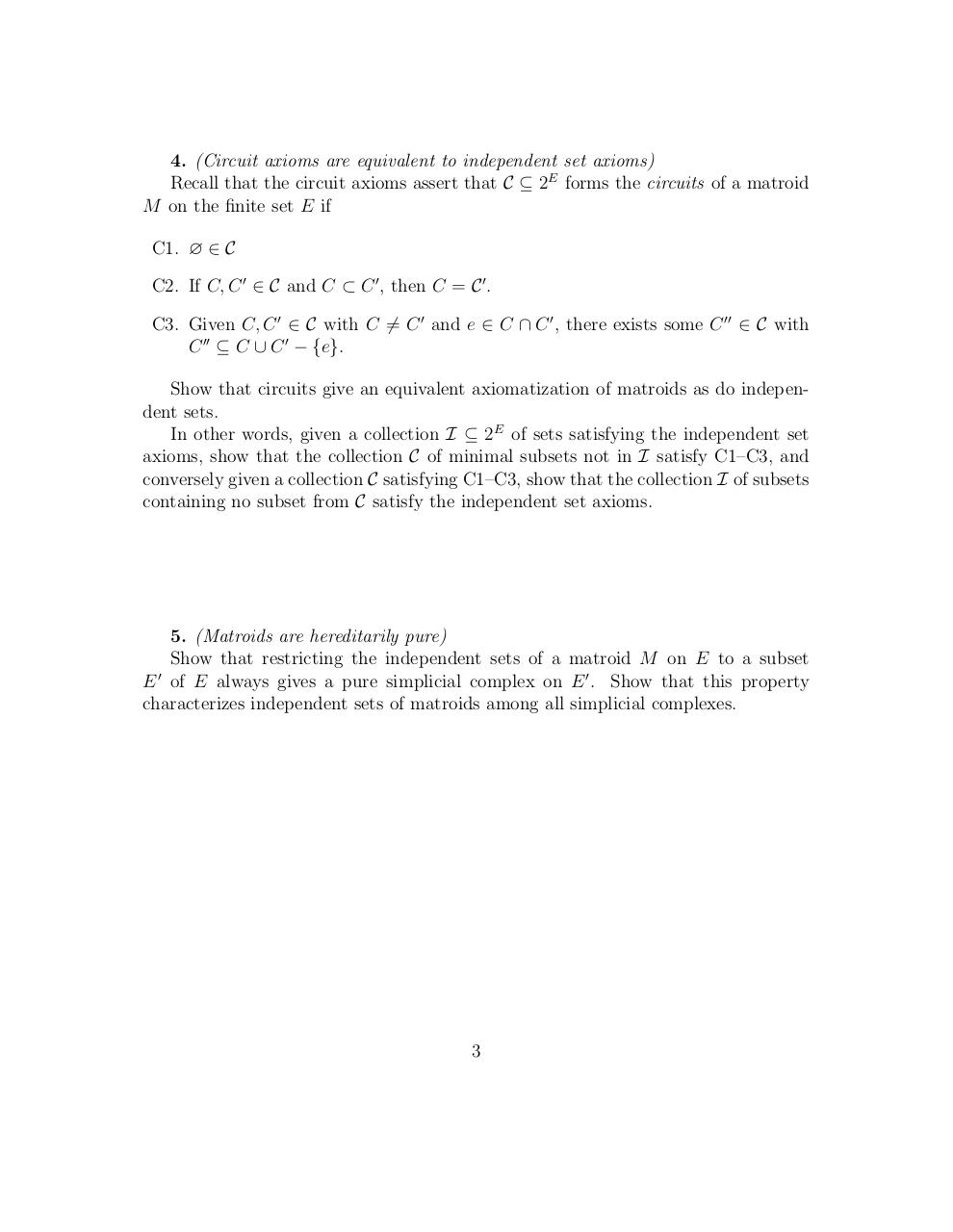# thing .pdf

### File information

Original filename: thing.pdf

This PDF 1.5 document has been generated by TeX / pdfTeX-1.40.16, and has been sent on pdf-archive.com on 27/06/2016 at 03:55, from IP address 66.253.x.x. The current document download page has been viewed 562 times.
File size: 108 KB (3 pages).
Privacy: public file

thing.pdf (PDF, 108 KB)

### Document preview

Lecture 1 Problems
1*.
(a) (Graphic matroids are linearly representable)
Given a graph G = (V, E), show that one can linearly represent its graphic
matroid over any field F as follows. In the vector space FV having standard
basis vectors i indexed by the vertices V in V , represent the element e = {v, v 0 }
in E by the vector v − v0 .
In other words, show that the linearly independent subsets of these vectors are
indexed by the forests of edges in G.
(b) (Transversal linearly representable)
Given a bipartite graph G with vertex bipartition E ∪ F , show that one can
represent its transversal matroid in any field characteristic as follows. Let F(xe,f )
be a field extension of the field F by transcendentals {xe,f } indexed by all edges
{e, f } of G. Then in the vector space F(xe,f )F having standard basis vectors f
indexed by the vertices f in F , represent the element e ∈ E by the vector
X
xe,f f .
f ∈F :{e,f }∈G

In other words, show that the linearly independent subsets of these vectors are
indexed by the subsets of vertices in E that can be matched into F along edges
of G.
(c) (Linearly representable are algebraically representable)
Given a matroid M of rank r linearly representd by a set of vectors {v1 , . . . , vn }
in the vector space Fr , represent M algebraically by elements of the rational
function field F(x1 , . . . , xr ) as follows. If vi has coordinates (vP
i1 , . . . , vir ) with
r
respect to the standard basis for F , then represent vi by fi := ri=1 vij xj .
In other words, show that the algebraically independent subsets of these rational
functions fi are indexed the same as the linearly independent subsets of the vi .

1

2*. (Acyclic orientations and chambers)
For a graph G = (V, E), consider the hyperplane arrangement A having hyperplanes of the form {xi = xj }{i,j}∈E . Explain how each top-dimensional cell (or
chamber or region) in the decomposition of RV cut out by the hyperplanes is naturally labelled by an acyclic orientation of the edges of G, and why this gives a
bijection between the chambers and the acylic orientations.

3*. (The greedy algorithm works for matroids)
(a) Show that Kruskal’s greedy algorithm (described in Lecture 1) always finds a
maximum-weight independent set in a matroid, regardless of the choice of weight
function w : E → R+ .
(b) Show that this property characterizes independent sets of matroids among all
simplicial complexes. In other words, given a simplicial complex I for which the
greedy algorithm always works, regarless of the weight function w, show that
I = I(M ) for a matroid M .
(Hint: One only needs to show that the exchange axiom I3 holds. To do this,
given I1 , I2 in I, with |I2 | = |I1 | + 1 = k + 1, consider the weight function
 k+1
for e ∈ I1
k+2
.
w(e) :=
k
for e ∈ I2 − I1
k+1
Explain why the greedy algorithm will build up I1 first, and then at the next
step, will exhibit an element of the form I1 ∪ {e} ∈ I with e ∈ I2 − I1 . In
particular, explain why the algorithm will not just stop after having found I1 !)

2

4. (Circuit axioms are equivalent to independent set axioms)
Recall that the circuit axioms assert that C ⊆ 2E forms the circuits of a matroid
M on the finite set E if
C1. ∅ ∈ C
C2. If C, C 0 ∈ C and C ⊂ C 0 , then C = C 0 .
C3. Given C, C 0 ∈ C with C 6= C 0 and e ∈ C ∩ C 0 , there exists some C 00 ∈ C with
C 00 ⊆ C ∪ C 0 − {e}.
Show that circuits give an equivalent axiomatization of matroids as do independent sets.
In other words, given a collection I ⊆ 2E of sets satisfying the independent set
axioms, show that the collection C of minimal subsets not in I satisfy C1–C3, and
conversely given a collection C satisfying C1–C3, show that the collection I of subsets
containing no subset from C satisfy the independent set axioms.

5. (Matroids are hereditarily pure)
Show that restricting the independent sets of a matroid M on E to a subset
0
E of E always gives a pure simplicial complex on E 0 . Show that this property
characterizes independent sets of matroids among all simplicial complexes.

3#### HTML Code

Copy the following HTML code to share your document on a Website or Blog

#### QR Code### Related keywords